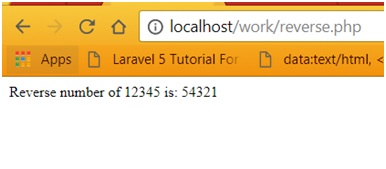# PHP code to reverse an integer number

Here, we will learn how to reverse an integer number in PHP? This post contains PHP code with output and explanation.
Submitted by IncludeHelp, on October 15, 2017

Given an integer number and we have to find, print its reverse number using PHP.

Example

```Input number is: 12345
Reverse number will be: 54321
```

Logic

• Take (input or assign) a number
• Assign 0 to the variable, in which we are going to store reverse number (Here, I am using ‘reverse’)
• Run the following two steps until number is not zero
• Get the digit using modules (%) operator and add it into the ‘reverse*10’
• Now, divide the number by 10
• Finally, when number will be zero, print the ‘reverse’

PHP code to reverse an integer number

```<?php
//input
\$num = 12345;
//reverse should be 0
\$reverse = 0;
//run loop until num is not zero
while (\$num != 0)
{
\$reverse = \$reverse * 10 + \$num % 10;
\$num = (int)(\$num / 10);
}
/printing the reverse number
echo "Reverse number of 12345 is: \$reverse";
?>
```

Output